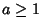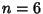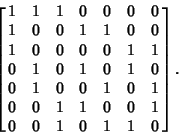## Projective Plane

A projective plane is derived from a usual Plane by addition of a Line at Infinity. A projective plane of orderis a set ofPoints with the properties that:

1. Any two Points determine a Line,

2. Any two Lines determine a Point,

3. Every Point hasLines on it, and

4. Every Line containsPoints.
(Note that some of these properties are redundant.) A projective plane is therefore a Symmetric (,, 1) Block Design. An Affine Plane of orderexists Iff a projective plane of orderexists.

A finite projective plane exists when the orderis a Power of a Prime, i.e.,for. It is conjectured that these are the only possible projective planes, but proving this remains one of the most important unsolved problems in Combinatorics. The first few orders which are not of this form are 6, 10, 12, 14, 15, ....

It has been proven analytically that there are no projective planes of order 6. By answering Lam's Problem in the negative using massive computer calculations on top of some mathematics, it has been proved that there are no finite projective planes of order 10 (Lam 1991). The status of the order 12 projective plane remains open. The remarkable Bruck-Ryser-Chowla Theorem says that if a projective plane of orderexists, andor 2 (mod 4), thenis the sum of two Squares. This rules out.

The projective plane of order 2, also known as the Fano Plane, is denoted PG(2, 2). It has Incidence MatrixEvery row and column contains 3 1s, and any pair of rows/columns has a single 1 in common.

The projective plane has Euler Characteristic 1, and the Heawood Conjecture therefore shows that any set of regions on it can be colored using six colors only (Saaty 1986).

See also Affine Plane, Bruck-Ryser-Chowla Theorem, Fano Plane, Lam's Problem, Map Coloring, Moufang Plane, Projective Plane PK2, Real Projective Plane

References

Ball, W. W. R. and Coxeter, H. S. M. Mathematical Recreations and Essays, 13th ed. New York: Dover, pp. 281-287, 1987.

Lam, C. W. H. The Search for a Finite Projective Plane of Order 10.'' Amer. Math. Monthly 98, 305-318, 1991.

Lindner, C. C. and Rodger, C. A. Design Theory. Boca Raton, FL: CRC Press, 1997.

Pinkall, U. Models of the Real Projective Plane.'' Ch. 6 in Mathematical Models from the Collections of Universities and Museums (Ed. G. Fischer). Braunschweig, Germany: Vieweg, pp. 63-67, 1986.

Saaty, T. L. and Kainen, P. C. The Four-Color Problem: Assaults and Conquest. New York: Dover, p. 45, 1986.